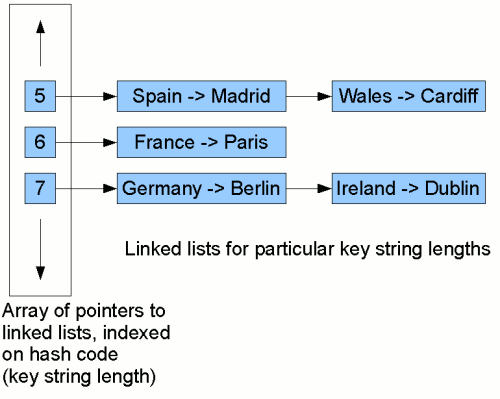## Java Collections: introduction to hashing and hash maps

On the previous page, we introduced the notion of hashing, mapping a piece of data such as a string to some kind of a representative integer value. We can then create a map by using this hash as an index into an array of key/value pairs. Such a structure is generally called a hash table or, particularly in Java parlance, hash map1. We saw that using the string length to create the hash, and indexing a simple array, could work in some restricted cases, but is no good generally: for example, we have the problem of collisions (several keys with the same length) and wasted space if a few keys are vastly larger than the majority.

### Buckets

Now, we can solve the problem of collisions by having an array of (references to) linked lists2 rather than simply an array of keys/values. Each little list is generally called a bucket.

Then, we can solve the problem of having an array that is too large simply by taking the hash code modulo a certain array size3. So for example, if the array were 32 positions in size, going from 0-31, then rather than storing a key/value pair in the list at position 33, we store it at position (33 mod 32) = 1. (In simple terms, we "wrap round" when we reach the end of the array.) So we end up with a structure something like this:Each node in the linked lists stores a pairing of a key with a value. Now, to look for the mapping for, say, Ireland, we first compute this key's hash code (in this case, the string length, 7). Then we start traversing the linked list at position 7 in the table. We traverse each node in the list, comparing the key stored in that node with Ireland. When we find a match, we return the value from the pair stored in that node (Dublin). In our example here, we find it on the second comparison. So although we have to do some comparisons, if the list at a given position in the table is fairly short, we'll still reduce significantly the amount of work we need to do to find a given key/value mapping.

The structure we have just illustrated is essentially the one used by Java's hash maps and hash sets. However, we generally wouldn't want to use the string length as the hash code. In the next sections, we'll explore how to generate more adequate hash codes.

## Improving our hash function

The method that we use to turn an object into a hash code is called the hash function. We'll see on the next page that rather than using the string length, we need to use a more adequate hash function.

Notes:
1. In the Java community, this double nomenclature has come about for historic reasons. Originally, Java had the class Hashtable with all-synchronized methods. When the Java Collections framework was introduced, Hashtable was effectively replaced with the HashMap class. As with collections classes in general, this class has unsynchronized methods, and via the method Collections.synchronizedMap() can be "wrapped" in a synchronized accessors.
2. This is the way Java hash maps solve the problem and is the avenue we will explore for the remainder of this tutorial. Another method sometimes used is to store colliding keys/values in positions in the array adjacent to where they would otherwise have gone. This of course requires that the array is big enough to hold as many mappings as will be added.
3. In other words, we divide the hash code by the array size and use the remainder as the array position.

If you enjoy this Java programming article, please share with friends and colleagues. Follow the author on Twitter for the latest news and rants.